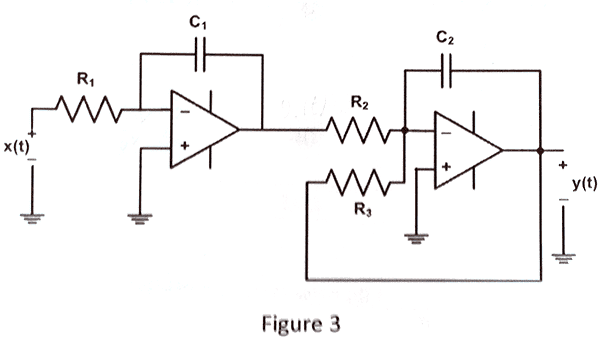# How to derive equation from op amp circuit?

• Engineering
• Nat3
In summary, the conversation discusses deriving a differential equation from a circuit and using Laplace transform for solving it. The suggested equation is y'' + y'/T2 = kx/T1T2 and the dc gain for the second stage is k.

## Homework Statement

Derive the differential equation from the following circuit## Homework Equations

The solution needs to be a differential equation in standard form, so that I can solve the equation for the characteristic modes, zero-input response, zero-state response, etc.

## The Attempt at a Solution

For the first op amp, I did this:

$\frac{x(t)-0}{R_1} = C_1\frac{dV_{C_1}}{dt}$

$V_{C1} = 0 - y_1(t) = -y_1(t)$

$\frac{x(t)}{R_1} = C_1\frac{dy_1(t)}{dt}$

$\frac{dy_1(t)}{dt} = \frac{x(t)}{R_1C_1}$

Then, for the second op amp:

$\frac{y_1(t)}{R_2} = C_2\frac{dV_{C_2}}{dt} + \frac{0-y(t)}{R_3}$

$V_{C_2}=0-y(t)$

$\frac{y_1(t)}{R_2} = -C_2\frac{dy(t)}{dt} - \frac{y(t)}{R_3}$

At this point, I'm stuck.

Any help would be greatly appreciated!

Have you had the Laplace transform?

If not, solve for y1(t) and then write the d.e. for the second stage with y1(t) the input for the second stage.

So y2(t) is a d.e with y1(t) as the excitation function.

You did OK but insert y1(t) as part of your d.e. for y2(t) after you solved for y1(t).

•1 person

No Laplace transform yet..

I plugged the first equation into the second and got this:

$\frac{\int \frac{x(t)}{R_1C_1}}{R_2} = -C_2\frac{dy(t)}{dt}-\frac{y(t)}{R_3}$

Differentiating both sides results in:

$\frac{\frac{x(t)}{R_1C_1}}{R_2} = -C_2\frac{d^2y(t)}{dt^2}-\frac{1}{R_3}\frac{dy(t)}{dt}$

or

$\frac{x(t)}{R_1R_2C_1} = -C_2D^2y(t)-\frac{Dy(t)}{R_3}$

or

$D^2y(t)+\frac{Dy(t)}{R_3C_2} = -\frac{x(t)}{R_1R_2C_1C_2}$

Is that correct?

Nat3 said:
$D^2y(t)+\frac{Dy(t)}{R_3C_2} = -\frac{x(t)}{R_1R_2C_1C_2}$

Is that correct?

Yes, except for the - sign on the rhs.

There arer two inversions so the output is + if the input is +.

Suggestion: if you let R3/R2 = k , R1C1 = T1 and R3C2 = T2 the math is cleaner. The equation then is
y'' + y'/T2 = kx/T1T2.

If you look at the 2nd stage the dc gain is k.

When you get the Laplace transform all this becomes much easier.

•1 person